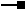ArcObjects Library Reference (GeoDatabase)

# INetWeightEdit Interface

Provides access to members that set information for this NetWeight object.

#### Product Availability

Available with ArcGIS Engine, ArcGIS Desktop, and ArcGIS Server.

#### When To Use

Use the INetWeightEdit interface for specifying parameters for a new weight to be added to a stand-alone logical network. When adding a weight to a logical network you can use this object to specify its WeightName, WeightType, and BitGateSize. A BitGateSize is only needed for bitgate weights.

#### Members

DescriptionBitGateSize Bit gate size of the network weight described by this NetWeight object.BitGateSize Bit gate size of this NetWeight object.WeightID Internal ID of the network weight described by this NetWeight object.WeightName Name of the network weight described by this NetWeight object.WeightName Name of this NetWeight object.WeightType Type of network weight described by this NetWeight object.WeightType Type of network weight of this NetWeight object.

#### Inherited Interfaces

Interfaces Description
INetWeight Provides access to members that get information about the network weight described by this NetWeight object.

#### CoClasses that implement INetWeightEdit

CoClasses and Classes Description
NetWeight A container for defining a weight on the network.

#### Remarks

To add this weight to your logical network you need to use INetSchemaEdit::AddWeight .

The INetWeightEdit interface is only for adding weights to a stand-alone logical network. For creating weights when building a geometric network, use the Build Geometric Network Wizard, or the AddWeight method on the NetworkLoader object.

[C#]

You can create a new weight through the following code:

``INetWeight netWeight = new NetWeight();INetWeightEdit netWeightEdit = (INetWeightEdit)netWeight;netWeightEdit.WeightName_2 = "MyWeight";netWeightEdit.WeightType_2 = esriWeightType.esriWTInteger;``

If you want to create a bitgate weight there is one more parameter you need to specify. The following code shows you how to create a bitgate weight.

``INetWeight netWeight = new NetWeight();INetWeightEdit netWeightEdit = (INetWeightEdit)netWeight;netWeightEdit.WeightName_2 = "MyWeight";netWeightEdit.WeightType_2 = esriWeightType.esriWTBitGate;netWeightEdit.BitGateSize_2 = 10;``
[Visual Basic .NET]

You can create a new weight through the following code:

``Dim netWeight As INetWeight = New NetWeight()Dim netWeightEdit As INetWeightEdit = CType(netWeight, INetWeightEdit)netWeightEdit.WeightName_2 = "MyWeight"netWeightEdit.WeightType_2 = esriWeightType.esriWTInteger``

If you want to create a bitgate weight there is one more parameter you need to specify. The following code shows you how to create a bitgate weight.

``Dim netWeight As INetWeight = New NetWeight()Dim netWeightEdit As INetWeightEdit = CType(netWeight, INetWeightEdit)netWeightEdit.WeightName_2 = "MyWeight"netWeightEdit.WeightType_2 = esriWeightType.esriWTBitGatenetWeightEdit.BitGateSize_2 = 10``